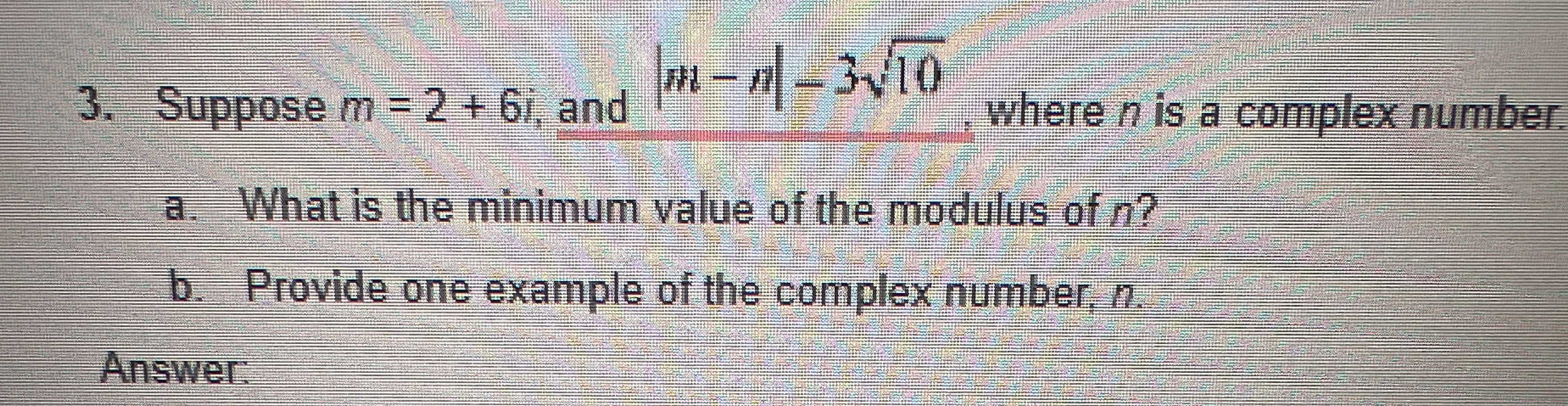### Still have math questions?

Arithmetic
Question3. Suppose $$m = 2 + 6 i$$ , and $$| m - n | - 3 \sqrt { 10 }$$ , where $$n$$ is a complex number. a. What is the minimum value of the modulus of $$n$$ ? b. Provide one example of the complex number, $$n$$ . Answer.

Step-by-step explanation: Suppose $$m = 2 + 6 i$$ , and $$| m + n | = 3 \sqrt { 1 } 0$$

The modulus sign means $$m + n$$ can either be positive or negative as shown. If it is positive:.2+6i+n $$= 3 \sqrt { 1 } 0$$

$$n = 3 \sqrt { 1 } 0 - ( 2 + 6 i )$$

$$n = 3 \sqrt { 1 } 0 - 2 - 18 \sqrt { 1 } 0 i$$

$$n = ( - 2 + 3 \sqrt { 1 } 0 ) + \sqrt { 1 } 0 i$$

b) Example of the complex number is given as $$( - 2 + 3 \sqrt { 1 } 0 ) + \sqrt { 1 } 0 i$$ . This is a complex number because it contains the real part and the imaginary part.

Solution
View full explanation on CameraMath App.